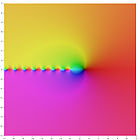# 多伽玛函数

${\boldsymbol {m}}$阶多伽玛函数伽玛函数的第$({\boldsymbol {m+1}})$对数导数

$\psi ^{(m)}(\zeta )=\left({\frac {d}{d\zeta }}\right)^{m}\psi (\zeta )=\left({\frac {d}{d\zeta }}\right)^{m+1}\ln \Gamma (\zeta )$$\psi (\zeta )=\psi ^{(0)}(\zeta )={\frac {\Gamma '(\zeta )}{\Gamma (\zeta )}}$$\ln \Gamma (\zeta )\!$$\psi ^{(0)}(\zeta )\!$$\psi ^{(1)}(\zeta )\!$$\psi ^{(2)}(\zeta )\!$$\psi ^{(3)}(\zeta )\!$$\psi ^{(4)}(\zeta )\!$## 积分表示法

$\psi ^{(m)}(\zeta )=(-1)^{m+1}\int _{0}^{\infty }{\frac {t^{m}e^{-\zeta t}}{1-e^{-t}}}dt$## 递推关系

$\psi ^{(m)}(z+1)=\psi ^{(m)}(z)+(-1)^{m}\;m!\;z^{-(m+1)}.$## 乘法定理

$k^{m}\psi ^{(m-1)}(kz)=\sum _{n=0}^{k-1}\psi ^{(m-1)}\left(z+{\frac {n}{k}}\right)$$k(\psi (kz)-\log(k))=\sum _{n=0}^{k-1}\psi \left(z+{\frac {n}{k}}\right)$## 级数表示法

$\psi ^{(m)}(z)=(-1)^{m+1}\;m!\;\sum _{k=0}^{\infty }{\frac {1}{(z+k)^{m+1}}}$m > 0和任何不等于负数的复数z都成立。还可以用赫尔维茨ζ函数来表示：

$\psi ^{(m)}(z)=(-1)^{m+1}\;m!\;\zeta (m+1,z).$## 泰勒级数

z = 1时，泰勒级数为：

$\psi ^{(m)}(z+1)=\sum _{k=0}^{\infty }(-1)^{m+k+1}(m+k)!\;\zeta (m+k+1)\;{\frac {z^{k}}{k!}},$